# Bioelectrical Impedance Analysis in Monitoring of the Clinical Status and Diagnosis of Diseases

#### By Cosimo Carriero

Field Applications Engineer

Analog Devices

October 07, 2019

Blog

## Bioelectrical impedance analysis is a low cost, noninvasive technique for measuring the composition of the human body and evaluating clinical conditions.

The electrical properties of biological tissues are classified as active or passive depending on the source of electricity. We talk about active response when biological tissues generate electricity due to the ions inside the cells. These electrical signals are referred to as biopotentials and the best known examples can be found in electrocardiography and electroencephalography. The response is passive when the biological tissue responds to an external electrical stimulus, like a current or a voltage generator. In this case, we are dealing with a bioimpedance.

## Bioelectrical Impedance Analysis

Bioelectrical impedance analysis is a low cost, noninvasive technique for measuring the composition of the human body and evaluating clinical conditions. Biological impedance is a complex quantity composed of a resistive value R (real part), mainly due to the total value of water in the body, and a reactive value Xc (imaginary part), mainly due to the capacitance created by the cell membrane. The impedance can also be represented as a vector, with module | Z | and phase angle φ. The phase angle plays a fundamental role in determining the composition of the body.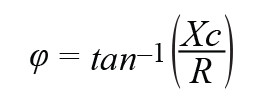The resistance R of a conductor of cross-sectional area S and length l and the capacitance C of a flat parallel plate capacitor with surface area S at distance d are given by the following equations: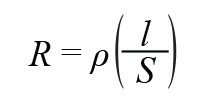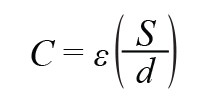As can be seen from Equation 4 and Equation 5, resistance and capacitance depend on geometrical parameters (length, distance, and surface area), which means they are linked to the adopted measurement system, and physical parameters; that is, the resistivity ρ and the dielectric constant ε, which are closely related to the type of material (in this case, the biological tissue) to be measured. Figure 1 shows a simplified electrical model of bioimpedance and of the instrument used to measure it. RE takes into account the resistance of extracellular fluids, RI symbolizes the resistance of intracellular fluids, and Cm is the capacitance of the cell membrane. The connection between the instrument and the human body occurs through electrodes applied to the skin. The instrument supplies the excitation voltage to the electrodes and measures the current produced. The excitation signal is generated by means of a digital-to-analog converter (DAC) connected to a downstream driver; the DAC is programmed by a microcontroller to enable the setting of the amplitude and the frequency of the signal. For the current measurement, a transimpedance amplifier (TIA) is used, connected to a high resolution analog-to-digital converter (ADC) for precise measurements. The acquired data are processed by the system microcontroller, which extracts the information required for the analysis.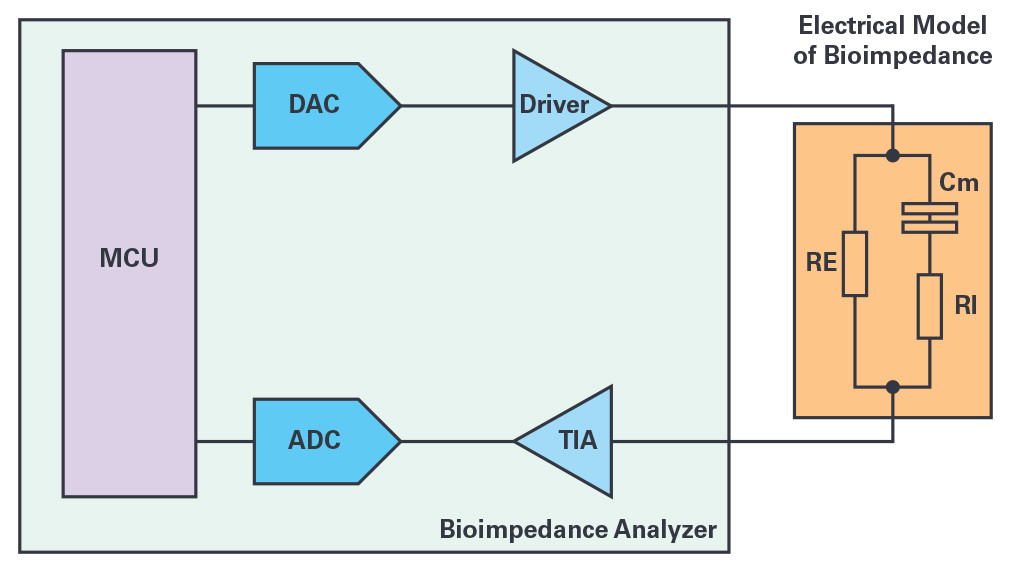Figure 1. Block diagram of the bioimpedance measurement system.

For bioimpedance measurements, the human body is divided into five segments: the two upper limbs, the two lower limbs, and the torso. This distinction is important for understanding the measurement method used. The most common are hand-to-foot, foot-to-foot, and hand-to-hand.

There are multiple factors to be taken into account during a bioelectrical impedance analysis (BIA) test, including anthropometric parameters; that is, height, weight, thickness of the skin, and build. Other factors are sex, age, ethnic group, and—not least—the patient’s state of health; that is, any malnutrition or dehydration. If these factors are not taken into consideration, the test results could be distorted. The interpretation of the measurements is based on statistical data and equations that take these various factors into account.

## Composition of the Human Body

When studying body composition, we refer to the three-compartment model, which includes the following:

• Fat mass
• Cell mass
• Extracellular mass

Figure 2 illustrates these concepts starting from the well-known terms of lean mass (fat-free mass) and fat mass of the two-compartment model. The fat mass has two components, the essential fat and the storage fat. The lean mass is divided into the body cell mass, composed of protein mass and intracellular water, and the extracellular mass, which in turn includes extracellular water and bone mass. A final parameter, fundamental to establishing the degree of hydration, is the total body water given by the sum of the intracellular and extracellular water.

From an electrical point of view, intracellular and extracellular electrolytic solutions behave like good conductors, whereas fat and bone tissue are poor conductors.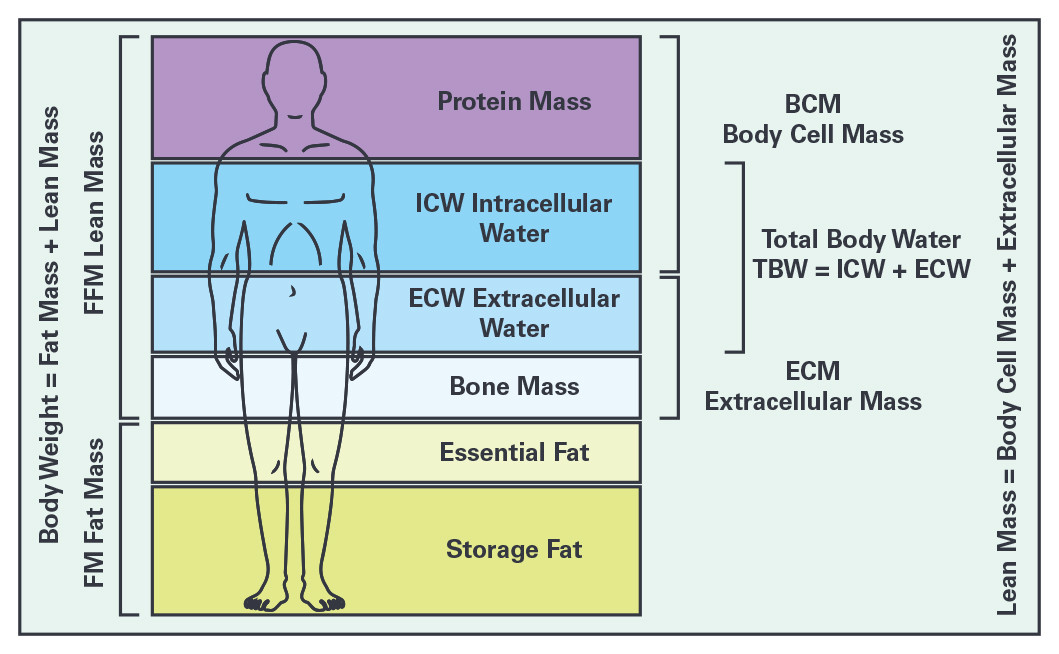Figure 2. Composition of the human body.

## Bioimpedance Measurement Techniques

The most widespread techniques for the measurement of bioimpedance differ in the use of the frequency of the excitation signal. The simplest instruments are based on measurements at a fixed frequency (single-frequency bioelectrical impedance analysis, or SF-BIA), some adopt a system with multiple frequencies (multifrequency bioelectrical impedance analysis, or MF-BIA), and the most sophisticated instruments perform real spectroscopy over a range of frequencies (bioimpedance spectroscopy, or BIS). There are also different techniques for the evaluation of the results, of which bioelectrical impedance vector analysis and real-time analysis are the most important.

In SF-BIA instruments, the current injected into the human body has a frequency of 50 kHz; the operation is based on the inversely proportional relationship between the measured impedance and the total body water (TBW)—the conductive part of the impedance–composed in turn of intracellular water (ICW) and extracellular water (ECW). This technique provides good results in subjects in normal hydration conditions, whereas it loses its validity in subjects with strongly altered hydration, above all due to a limited capacity to evaluate the variations in the ICW.

The MF-BIA technique overcomes the limitations of SF-BIA by performing the measurement at low and high frequencies. The low frequency measurement allows for a more accurate estimate of the ECW, whereas at the high frequency, an estimate of the TBW is obtained. The ICW is given by the difference between the two estimates. However, this technique is also not perfect and shows limitations in the estimation of body fluids in the elderly population affected by disease.

Finally, the BIS is based on the measurement of impedance at zero frequency, which, according to the model of Figure 1, is the resistance RE due to the extracellular fluids, and at infinite frequency, which is the parallel of RE with RI. At these two frequency extremes, the capacitance due to the cell membrane behaves like an open circuit or a short circuit. Intermediate frequency measurements provide information related to the capacitance value. BIS provides more detailed information than other techniques do, but, in this case, the measurement takes longer time.

Bioimpedance vector analysis (bioelectrical impedance vector analysis, or BIVA) is a human health assessment technique based on the absolute measurement of bioimpedance. It uses a graph that shows a vector representation of the impedance in which the value of the resistance is shown on the abscissa and the value of the capacitive reactance on the ordinate, both values being normalized with respect to the patient’s height. The method is based on the formulation of three tolerance ellipses: 50 percent, 75 percent and 95 percent. The tolerance ellipse of 50 percent defines the population with average body composition. Moving along the horizontal axis of the ellipse, individuals with a low percentage of lean mass are identified on the right and vice versa; that is, those with a high percentage of lean mass are identified on the left. Moving along the vertical axis identifies the level of hydration, with levels below the norm toward the top of the ellipses and levels above the norm in the lower part.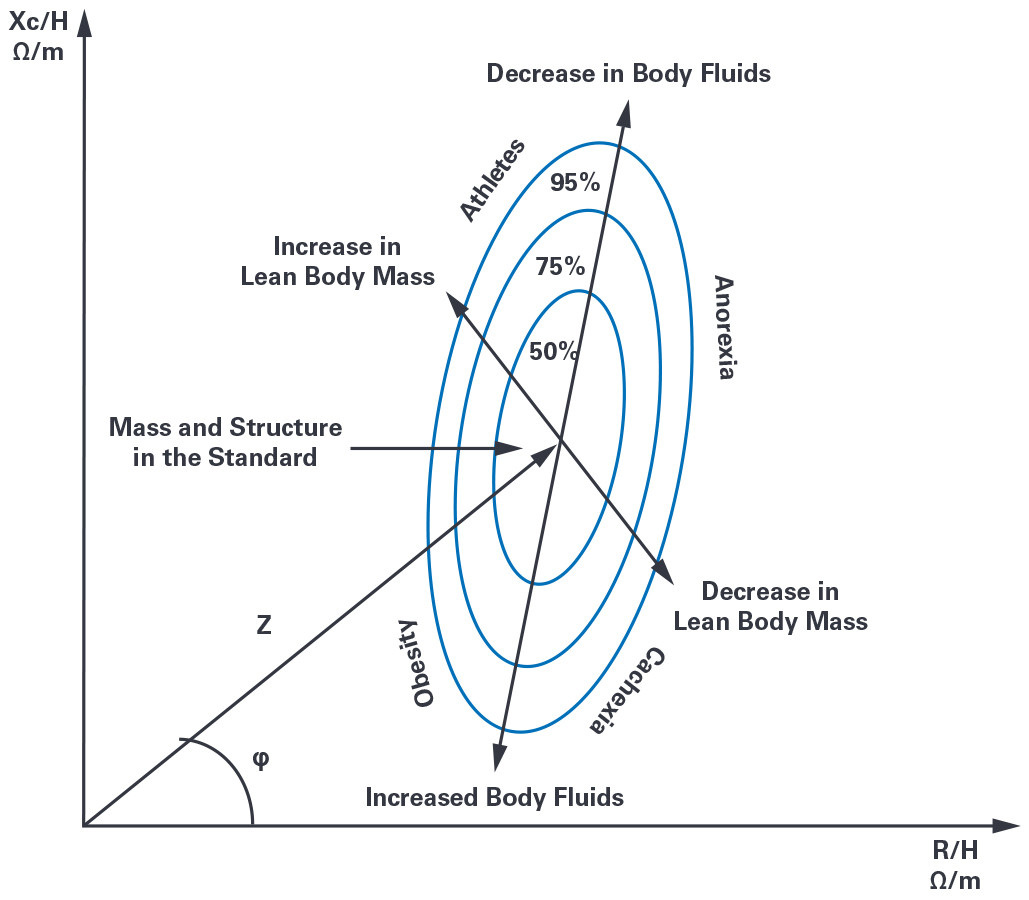Figure 3. Bioelectrical impedance vector—analysis tolerance ellipses.

The observation of fluctuations in the components of the human body—for example, the deviation from the normal values of the lean body mass, the fat mass, and the total body water–are key factors for establishing the state of health of the patient. A significant loss of lean mass and an imbalance of body fluids are the main parameters used for the diagnosis of diseases. Today, bioelectrical impedance analysis is used as an aid in the diagnosis of diseases of the following systems of the human body:

• Pulmonary system
• Lung cancer
• Pulmonary edema
• Cardiovascular system
• Accumulation of fluids after surgery
• Circulatory system
• Intravascular volume
• Hyponatremia
• Hydration
• Renal system
• Hemodialysis
• Evaluation of dry weight
• Nervous system
• Alzheimer’s disease
• Anorexia nervosa
• Muscular system
• Evolution of body composition during training
• Immune system
• Evaluations in HIV-infected patients
• Evaluations in cancer patients
• Dengue fever

## AD5940, a Flexible and High Precision Analog Front End

Analog Devices has a broad portfolio of products for impedance analysis, including devices such as the ADuCM35x, a highly integrated system on chip (SoC) designed specifically for impedance spectroscopy. Recently announced to the market, the AD5940 is a high precision, low power consumption analog front end, ideal for portable applications. Designed for the measurement of bioimpedance and skin conductivity, the AD5940 is composed of two excitation loops and a common measurement channel. The first excitation loop is able to generate signals with a maximum frequency of 200 Hz and can be configured as a potentiostat for the measurement of electrochemical cells of different types. The basic components are a dual-output DAC, a precision amplifier that provides the excitation signal, and a transimpedance amplifier for current measurement. Working at a low frequency, this loop consumes low power and is therefore also referred to as the low power loop. The second loop has a similar configuration but is able to work with signals up to 200 kHz; for this reason, it is called the high-speed loop. The device is equipped with an acquisition channel with a 16-bit, 800 kSPS SAR-type ADC and an analog signal processing chain upstream of the converter, which includes a buffer, a programmable gain amplifier (PGA), and a programmable antialiasing filter. To complete the architecture, there is a switching matrix mux that allows a multiplicity of signals coming from multiple internal or external sources to the device to be connected to the ADC. In this way, in addition to the primary impedance measurement function, accurate system diagnostics can be performed to verify the full functionality of the instrument.

Figure 4 shows the connection of the AD5940 for the absolute impedance measurement of the human body with a four-wire configuration. For this type of measurement, the high frequency loop is used; a programmable ac voltage generator provides the excitation signal. A second generator supplies the common-mode voltage—useful for a correct measurement. The current resulting from the impedance of the human body is measured by the transimpedance amplifier and converted with the 16-bit ADC. The system is able to measure up to a frequency of 200 kHz and provides a signal-to-noise ratio (SNR) of 100 dB at 50 kHz. The digital data are sent to a hardware accelerator for extraction of the quantities of interest; that is, the real part and the imaginary part of the impedance.

As a medical device, the bioimpedance analyzer must comply with the IEC 60601 standard. This standard sets limits for the voltages and currents that can be applied to the human body. For this reason, a resistance, Rlimit, has been provided to limit the maximum current and four coupling capacitors, CisoX, to prevent a dc component from being applied to the human body.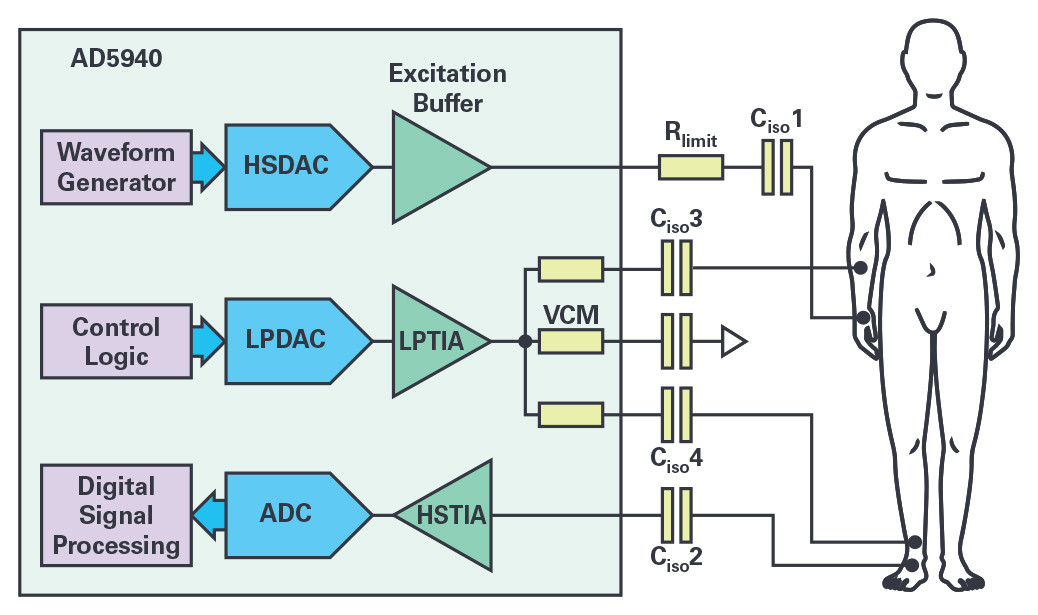Figure 4. Four-wire connection of the AD5940 for bioelectrical impedance analysis.

## Conclusion

Bioimpedance measurement is a versatile, fast, noninvasive, and low cost tool for assessing the composition of the human body and diagnosing certain types of diseases. Current technology, thanks to the use of devices such as the AD5940, enables the realization of compact, high performance, low power consumption bioimpedance analyzers that can be battery powered. The features of integration, small form factor, and low power consumption of the AD5940 also make it particularly suitable for wearable applications.